Courses

# RD Sharma Solutions -Ex-10.4, Congruent Triangles, Class 9, Maths Class 9 Notes | EduRev

## Class 9 : RD Sharma Solutions -Ex-10.4, Congruent Triangles, Class 9, Maths Class 9 Notes | EduRev

The document RD Sharma Solutions -Ex-10.4, Congruent Triangles, Class 9, Maths Class 9 Notes | EduRev is a part of the Class 9 Course RD Sharma Solutions for Class 9 Mathematics.
All you need of Class 9 at this link: Class 9

Q. (1) In fig (10).9(2) It is given that AB = CD and AD = BC. Prove that ΔADC≅ΔCBA.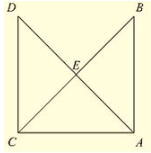Solution:

Given that in the figure AB = CD  and AD = BC.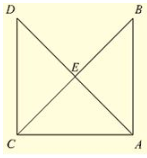Now,

Consider Δ ADC and Δ CBA.

We have

AB = CD                     [Given]

And AC = AC             [Common side]

So, by SSS congruence criterion, we have

Hence proved

Q. (2) In a Δ PQR. IF PQ = QR and L, M and N are the mid-points of the sides PQ, QR and RP respectively. Prove that LN = MN.

Sol: Given that in Δ PQR, PQ = QR and L, M and N are the mid-points of the sides PQ, QR and RP respectively

We have to prove LN = MN.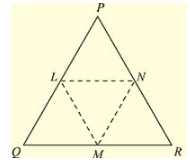Join L and M, M and N, N and L

We have PL = LQ, QM = MR and RN = NP

[Since, L, M and N are mid-points of Pp. QR and RP respectively]

And also PQ = QR

• PL = LQ = QM = MR =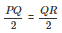……(i) Using mid-point theorem,

We have

MN ∥ PQ and MN =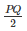• MN = PL = LQ ……(ii)

Similarly, we have

LN ∥  QR and LN = (1/2)QR

• LN = QM = MR ……(iii)

From equation (i), (ii) and (iii), we have

PL = LQ = QM = MR = MN = LN

LN = MN

Offer running on EduRev: Apply code STAYHOME200 to get INR 200 off on our premium plan EduRev Infinity!

91 docs

,

,

,

,

,

,

,

,

,

,

,

,

,

,

,

,

,

,

,

,

,

,

,

,

,

,

,

,

,

,

;